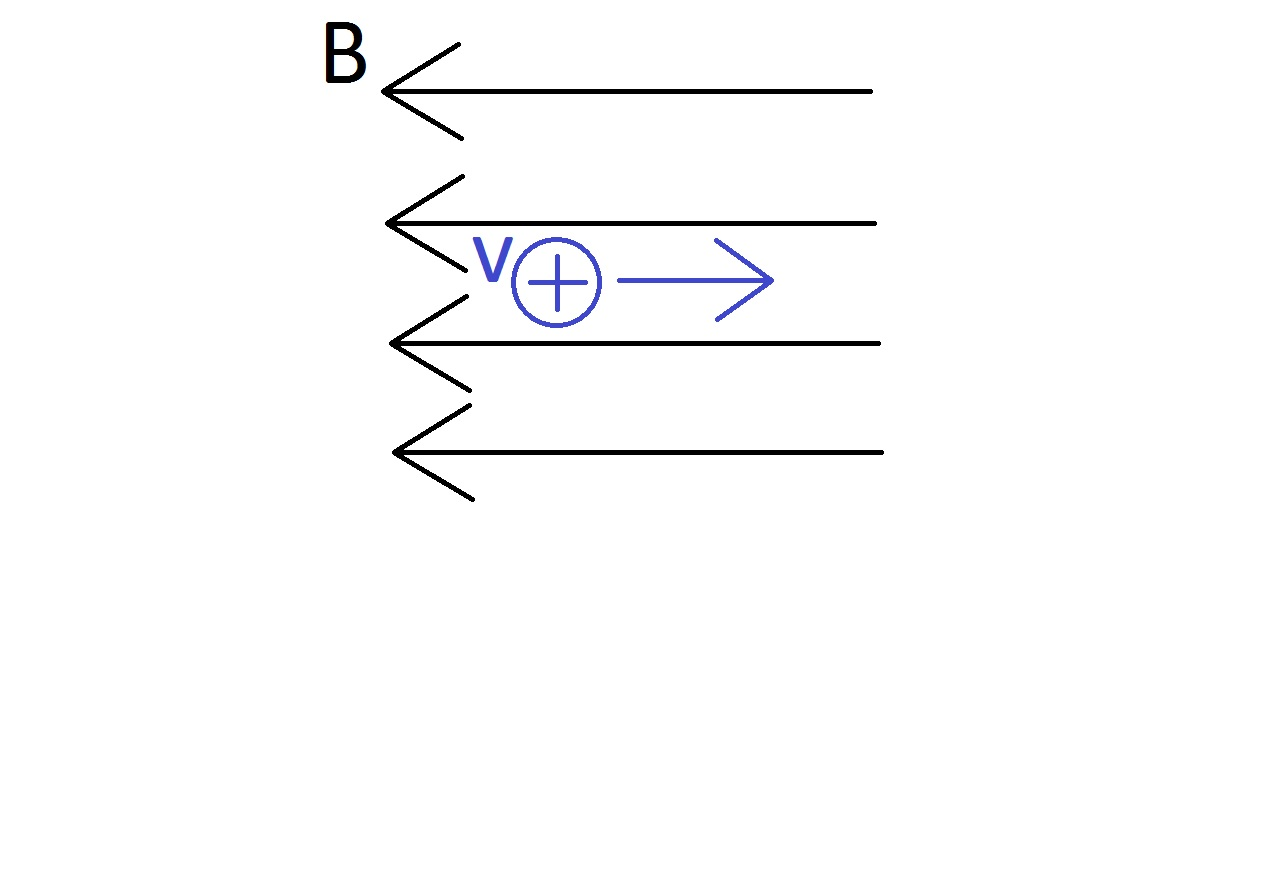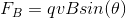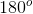College Physics : Magnetic Force

Example Questions

Example Question #1 : Electromagnetics

Suppose that a magnetic field is oriented such that it is pointing directly to the left, as in the picture shown below. If a positively charged particle were to begin traveling through this magnetic field to the right, in which direction would the particle's trajectory begin to curve?The particle would move into the page

The particle would move out of the page

The particle would move down the page

The particle would continue to move to the right unaffected

The particle would continue to move to the right unaffected

Explanation:

In order to answer this question, it's important to understand the factors that determine the magnetic force experienced by a charge. We can begin by writing out the equation for magnetic force.As shown in the above equation, the magnetic force is directly proportional to the particle's charge, its velocity, and the strength of the magnetic field itself. But, for the purposes of the this question, the most important factor is the angle of the particle's velocity with respect to the magnetic field.

Notice that if theta is equal to zero, then the sine of theta will be equal to zero as well. This, in turn, will cause the magnetic force to also be zero. This is also true if we were to define theta as.

Since the particle is moving in a direction that is parallel to the magnetic field lines but in the opposite direction, we have a situation in which theta is equal to. This means that the magnetic force on the particle is zero. As a result, the particle will continue to move through the magnetic field without changing its direction.

All College Physics Resources